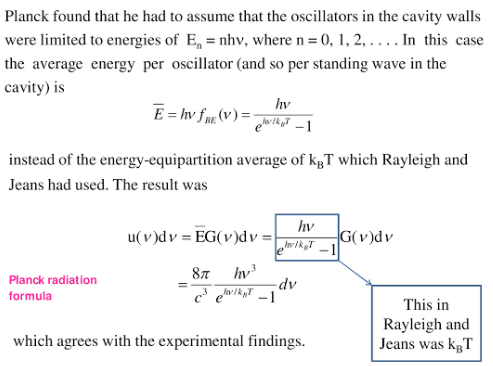Modern PhysicsRayleigh-Jeans law and Wien’s formulae were failed to solve the problem of distribution of spectral radiance among various wavelengths in cavity radiations because these formulas were based upon the classical theory.

## Planck’s quantum theory of radiation

In 1900, Max Planck gave a new concept about the nature of radiation, called the quantum theory of radiation in which Planck assumed the discrete nature of radiation. He assumed the atoms of the cavity emit and absorb radiation in the form of packets of energy, called quanta. The energy of each quantum is directly proportional to frequency.

E ∝ f
E =hf
where h is Planck’s constant.

• The atoms of the cavity behave like tiny harmonic oscillators.
• The oscillators radiate and absorb energy only in the form of packets or bundles of electromagnetic waves.
• An oscillator can emit or absorb any amount of energy which is the integral multiple of “hf ” which is mathematically expressed as:

E=nhf
Where n is an integer.